Polyhedron and round bodies1. The round bodies

The solid geometry studies the bodies that have three dimensions: length, width and height.
Bodies that have flat faces are called polyhedron.
The round bodies have a face that is a curved area.

There are three main kinds of round bodies: the cylinder, the sphere and the cone.Look at the three figures and answer these questions:

 Figure A is a...... Figure B is a...... Figure C is a......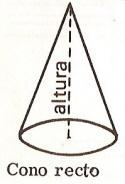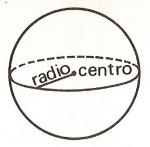2. Round bodies parts

The cylinder has always two bases. The distance between them (measured on a line that must be perpendicular to the bases) is called height.

The cone has a circular base and a point called apex or vertex. The distance from the vertex (measured on a line perpendicular to the base) is called height. The distance from the vertex to any point of the circumference of the base is called side of the cone.

The sphere has a special point called center which is equidistant from all points on the circle. The sphere has also radius and diameter.3. The prism

Geometric shapes having flat faces are called polyhedron.
The prism is a polyhedron that has as bases two equal polygons and each of its sides is a parallelogram.
Look at the picture and you will see that the triangular prism has a triangular base; the quadrangular, the quadrilateral, the pentagonal prism, the pentagon, the hexagonal, the hexagon and a square.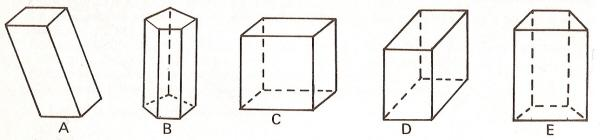The cube is formed by square faces; the parallelepiped is a prism whose faces are all parallelograms. The A and C are parallelepiped, the C is a cube, the B is a pentagonal and the E is quadrangular.

 The prism A is ... The prism B is ... ... The prism C is ... ... The prism D is ... The prism E is ...4 - The pyramid

The pyramid is a polyhedron whose base is any polygon and sides are triangles. The triangular sides meet at a point called the vertex or apex.
In the left picture, the point B is the vertex and the height is the BK segment. In the right picture, the vertex is the point A and the height is AH.
The tetrahedron is a pyramid composed of four triangular faces.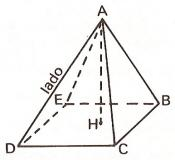The point A is a... The AB is a... The AH is a... The CD is a...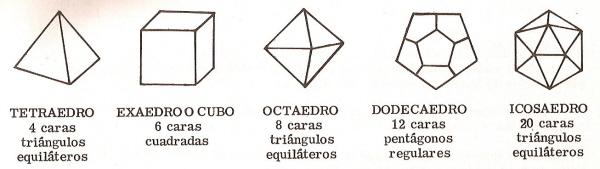5. Regular polyhedron

The regular polyhedron is composed of angles and faces that are all equal.
We have seen that the tetrahedron has 4 faces that are equilateral triangles. We have also seen the cube with 6 square faces. The other regular polygons are the octahedron, the dodecahedron and the icosahedron.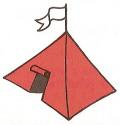Look at the five pictures and indicate what shape they have:

 The lamppost has... The hat is... The Chinese hat is... The prism is...... The store is a......5. How to build a regular polyhedron

To build the polyhedron of this graph, first draw each of the figures in a strong paper or cardboard and then cut it by the outlines. Then fold it along the dotted lines and join the edges. Apply glue on the external edges.

 .adslot_S1 { display:inline-block; width: 336px; height: 280px; } @media (max-device-width:970px) { .adslot_S1 { display: none; } } If you are registered, please enter your data:   Name:  Password:   | Educational applications | Mathematics | In Spanish  | To print

«Arturo Ramo GarcĒa.-Record of intellectual property of Teruel (Spain) No 141, of 29-IX-1999
Plaza Playa de Aro, 3, 1║ DO 44002-TERUEL